# 二分图学习笔记

## 定义

$$G=(V,E)$$是一个无向图，如果顶点$$V$$可分割为两个互不相交的子集$$(A,B)$$，并且图中的每条边$$（i，j）$$所关联的两个顶点$$i$$$$j$$分别属于这两个不同的顶点集$$(i \epsilon A,j\epsilon B)$$，则称图$$G$$为一个二分图。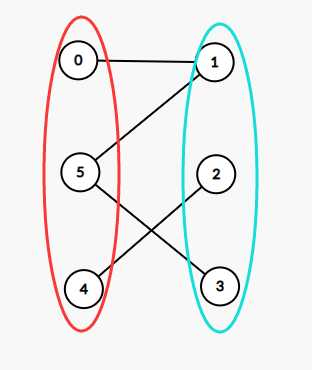### 二分图1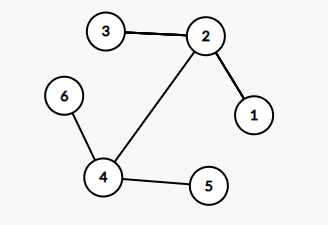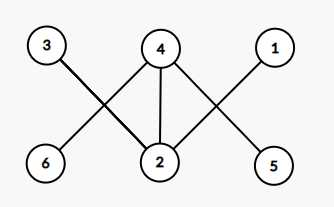### 二分图2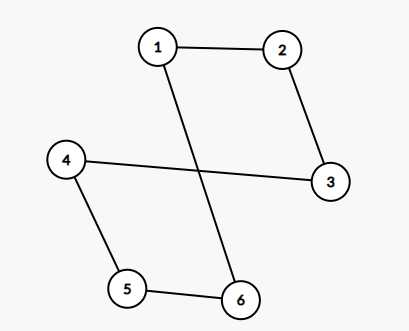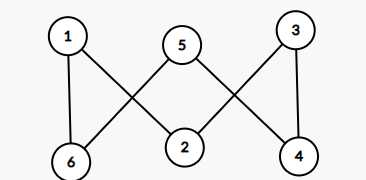### 二分图3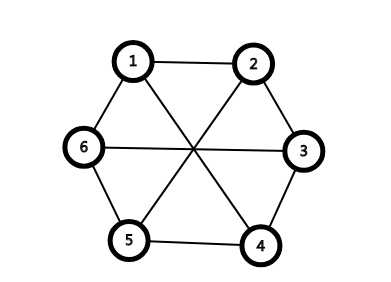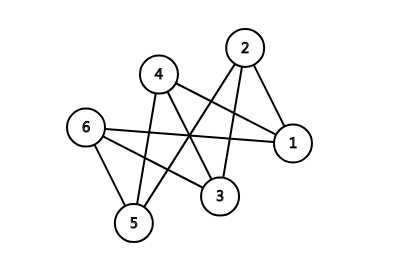## 性质

1. 如果两个集合中的点分别染成黑色和白色，可以发现二分图中的每一条边都一定是连接一个黑色点和一个白色点。
2. 二分图不存在长度为奇数的环，如果一个图是二分图，那么它一定没有奇环，如果一个图没有奇环的话，那么它可以是二分图

## 判定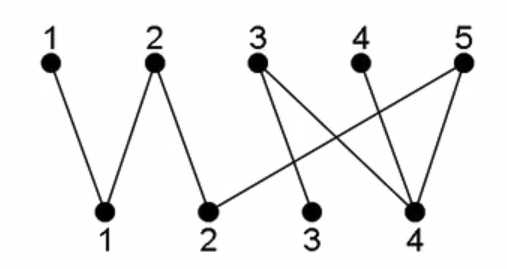## 相关概念

### 二分图最大匹配求法

1. 匈牙利算法 $$O(nm)$$
2. $$\text{HK}$$算法$$O(\sqrt{n}*m)$$
3. 网络最大流

### 二分图最佳匹配求法

1. $$\text{KM}$$算法（优化版$$O(n^3)$$
2. 网络最大流

## 匈牙利算法-求二分图最大匹配

### 由增广路的定义可以推出下述三个结论

1. $$P$$的路径长度必定为奇数，第一条边和最后一条边都不属于$$M$$
2. $$P$$经过取反操作可以得到一个更大的匹配$$M’$$
3. $$M$$$$G$$的最大匹配当且仅当不存在相对于$$M$$的增广路径。

### 算法轮廓：

1. $$M$$为空
2. 找出一条增广路径$$P$$,通过取反操作获得更大的匹配$$M’$$代替$$M$$
3. 重复$$2$$操作直到找不出增广路径为止

/*
Author：loceaner
*/
#include <cmath>
#include <cstdio>
#include <cstring>
#include <iostream>
#include <algorithm>
using namespace std;

const int A = 1e5 + 11;
const int B = 1e6 + 11;

char c = getchar(); int x = 0, f = 1;
for( ; !isdigit(c); c = getchar()) if(c == '-') f = -1;
for( ; isdigit(c); c = getchar()) x = x * 10 + (c ^ 48);
return x * f;
}

int n, m, match[A], E, vis[A], ans;
struct node { int to, nxt; } e[B];

inline void add(int from, int to) {
e[++cnt].to = to;
}

bool dfs(int now) {
for(int i = head[now]; i; i = e[i].nxt) {
int to = e[i].to;
if(vis[to]) continue;
vis[to] = 1;
if(!match[to] || dfs(match[to])) {
match[to] = now;
return 1;
}
}
return 0;
}

int main() {
for(int i = 1, x, y; i <= E; i++) {
if(x > n || y > m) continue;
}
for(int i = 1; i <= n; i++) {
memset(vis, 0, sizeof(vis));
if(dfs(i)) ans++;
}
cout << ans << '\n';
return 0;
}

## 其他相关概念

### 二分图最大独立集

(0)
(0)